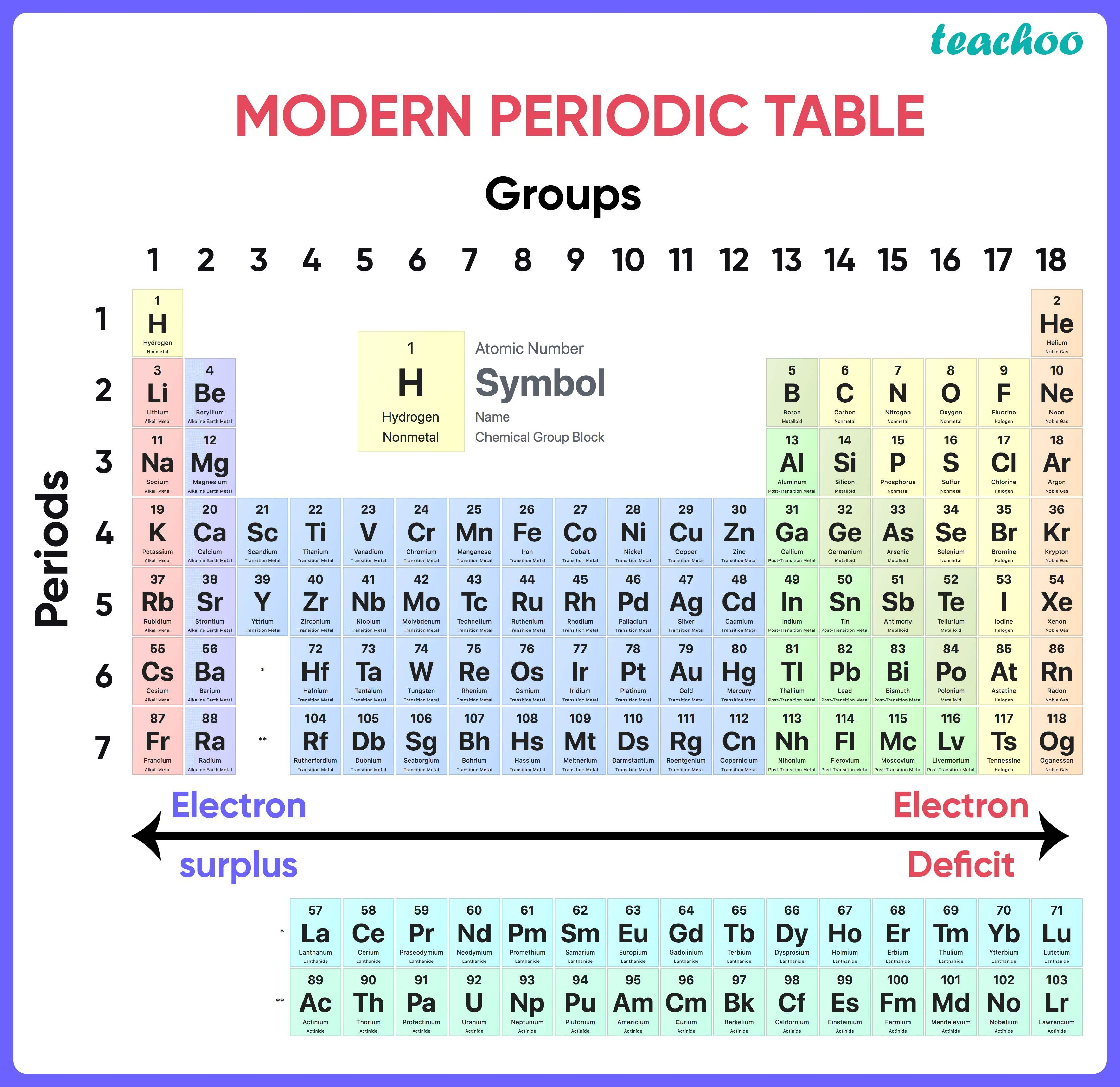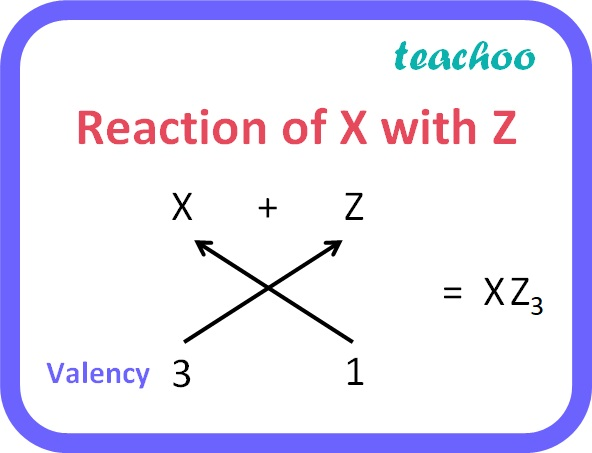Past Year - 3 Mark Questions

Class 10
Chapter 5 Class 10 - Periodic Classification of Elements

## Three elements ‘X’, ‘Y’ and ‘Z’ have atomic numbers 7, 8 and 9 respectively.

(a)

 X Y Z Atomic number of X = 7 Electronic configuration: 2, 5 Group number = 10 + number of electrons in outermost shell=10 + 5 = 15 Since there are 2 shells, Period = 2nd X is nitrogen Atomic number of Y = 8 Electronic configuration: 2, 6 Group number = 10 + number of electrons in outermost shell=10 + 6 = 16 Since there are 2 shells, Period=2nd Y is oxygen Atomic number of Z = 9 Electronic configuration: 2, 7 Group number = 10 + number of electrons in outermost shell=10 + 7 = 17 Since there are 2 shells, Period=2nd Z is Fluorine

(b)As we move across a period from left to right, atomic radii decreases. X, Y and Z belong to the same period ie., 2 and in the modern periodic table, X comes to the left of Y and Z comes to the right of Y . So the atomic radius of X is more than Y and the atomic radius of Z is less than Y.

So,the order of their atomic radii is: X>Y>Z

(c)

Valency of X = 8 - 5 = 3

Valency of Z = 8 - 7 = 1X reacts with Z to form XZ 3

Learn in your speed, with individual attention - Teachoo Maths 1-on-1 Class

### Transcript

Reaction of X with Z Valency